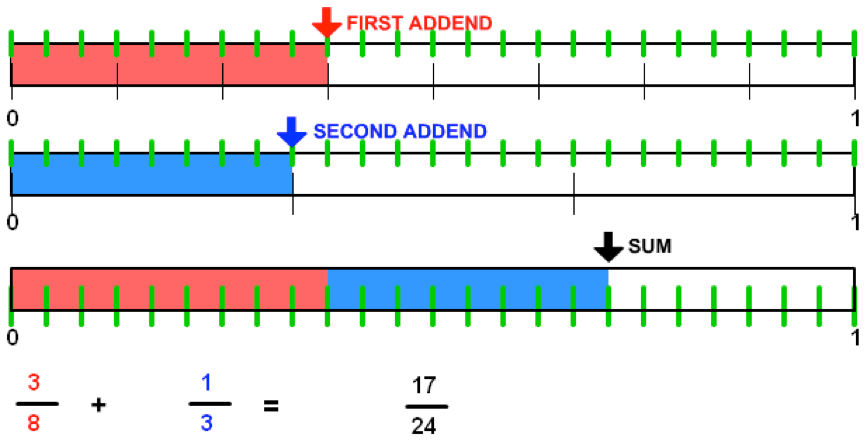# Adding and Subtracting Fractions with Number Lines

Number lines can be used to find equivalent fractions of the same denominator for two fractions with unlike denominators such as  $$\dfrac{2}{3}$$ and $$\dfrac{1}{4}$$.

The picture below illustrates $$\dfrac{3}{8}+\dfrac{1}{3}$$. The common denominator for $$8$$ and $$3$$ is $$24$$. The green tick marks divide the whole into $$24$$ths.

The bottom number line shows the addition of the two fractions with the whole divided into $$24$$ths.$\dfrac{3}{8}+\dfrac{1}{3}=\dfrac{17}{24}$

1. How many number lines were used? Why?
2. How were the number lines partitioned?
3. Use multiple number lines to add: $$\dfrac{1}{3}+\dfrac{1}{6}$$
4. Show how the same number line strategy will work to subtract: $$\dfrac{3}{8}-\dfrac{1}{3}$$

Check solutions here.